# Chapter 2. Oscillator Waveform Modification

Most of the oscillators in the Nord Modular provide only the most basic waveform shapes: Sine, Triangle, Sawtooth, and Square (or Pulse). The Formant oscillator and the Spectral Oscillator are exceptions to this, and we will discuss them later.

The so-called subtractive approach to sound synthesis uses filtering of these basic waveforms to provide control over the harmonic content of a sound. A sinewave has only a single harmonic, and therefore filtering does not alter its harmonic content at all, merely the amplitude of the sound. A triangle wave has more harmonics, but with very low amplitudes, so they too are not too useful as sources for subtractive synthesis. The most useful waveforms for subtractive synthesis are the sawtooth and square (or pulse) waveforms. These waveforms have strong harmonics, suitable for alteration through filtering. A sawtooth waveform contains all harmonics of the fundamental pitch of the waveform, while the square waveform contains just the odd harmonics. A pulse waveform, with a duty cycle different than 50% also has all harmonics, even and odd. Changing the pulse width alters the relative strength of the individual harmonics, with the harmonic levels become more equal as the pulse narrows.

In the subtractive synthesis approach, variations in the spectrum of the sound (or timbre) are usually generated by varying the characteristics, such as cutoff frequency and resonance, of the filters that the oscillator waveforms are passed through. While this is effective for synthesizing many pleasing and interesting sounds, the result is limited somewhat by the rather sparse choices of spectra for the oscillators. Because of this, synthesizer manufacturers and patch programmers have come up with a number of ways in which to expand the spectral possibilities beyond those of the basic oscillator waveforms. In this chapter we will examine some of these approaches and show how they can be implemented in the Nord Modular.

## 2.1 Oscillator Sync

Oscillator Sync alters the waveform of an oscillator by resetting the phase of the oscillator waveform when a sync pulse is received. If this sync pulse occurs at a rate faster than the nominal oscillator frequency, the oscillator will become locked to the sync rate. The specific harmonic structure of the synced oscillator waveform will depend on the nominal oscillator frequency. If the sync pulse occurs at a rate slower than the nominal oscillator frequency, the oscillator will not become locked to the sync rate, but will gain strong harmonic components corresponding to the sync frequency. I will not go into too much additional detail here, but instead refer you to the excellent tutorial on Oscillator Sync by Rob Hordijk, found on the Clavia web site at www.clavia.se/nordmodular/Modularzone/Hardsync.html and www.clavia.se/nordmodular/Modularzone/Softsync.html.

I will give here a soft-sync technique that was not covered in the tutorial. This technique was used in the RMS (Rivera Music Systems) synthesizer as well as in the Wiard synthesizers. In this technique, the synced oscillator produces a triangle wave (which could be passed through a wave shaper to produce other waveforms). To this triangle waveform a narrow pulse from the syncing oscillator is added. The sum is compared to a reference level. If the sum exceeds this reference level, the synced oscillator is reset, or the triangle wave reversed. The probability of a syncronization event being created can be seen to be related to the amplitude of the syncing pulse as well as the amplitude of the triangle wave output of the synced oscillator. If the syncing pulse has a low amplitude, then syncing will occur only if the syncing pulse happens very close in time to the peak of the triangle waveform. Otherwise no syncing will occur. At the other extreme, if the syncing pulse has a very large amplitude, then syncing will occur on every pulse, no matter what the phase of the triangle waveform. In this case, the sync action will be that of regular hard-sync. By adjusting the amplitude of the syncing pulse one can vary the sync effect from soft to hard. This technique is illustrated by the following Nord Modular patch. It is not exactly as in the RMS and Wiard synths, since the triangle wave is reset on reception of a sync signal, rather than being reversed. But for those intrepid patchers who want more authenticity, follow the instructions in Rob Hordijk's above referenced softsync tutorial, where he describes how to reverse the slope of a triangle waveform on the Nord Modular.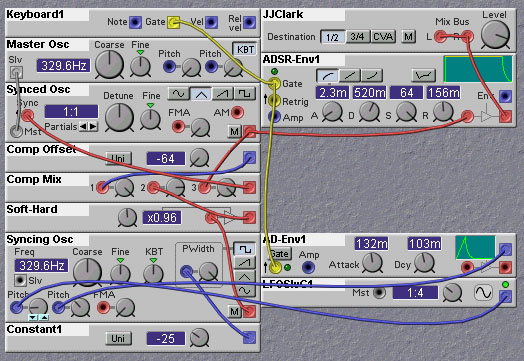Figure 2.1. Patch illustrating a softsync technique (J. Clark).

For the technical minded among you, there are some ways to make sync patches sound even better. The NM, as we all know, is a digital system at heart, even though it might sound "analog" from time to time. Because of the finite sampling rate used in the NM, the syncing waveform is actually quantized in time. This means that the synced oscillator is not only synced to the syncing waveform but also to the sampling clock. At low frequencies of the syncing waveform the effect of the sampling clock sync will not be too noticeable, but at high frequencies it becomes quite significant. Rob Hordijk suggests a way to remedy this problem. The jitter caused by the syncing to the sampling clock is most noticeable at the transient that inevitably appears at the point where the syncing signal causes the synced waveform to switch. One can apply a mask, or envelope, over the synced oscillator waveform, to suppress this transient. A simple way to do this is to use a downward sawtooth as the syncing waveform and also use it as an amplitude envelope that modulates the synced waveform. This has the drawback of distorting the synced waveform, of course. An improvement can be had by crossfading two such synced waveforms, each out of phase with respect to each other. This will minimize the distorting effect of the masking. The following patch by Rob Hordijk illustrates the approach.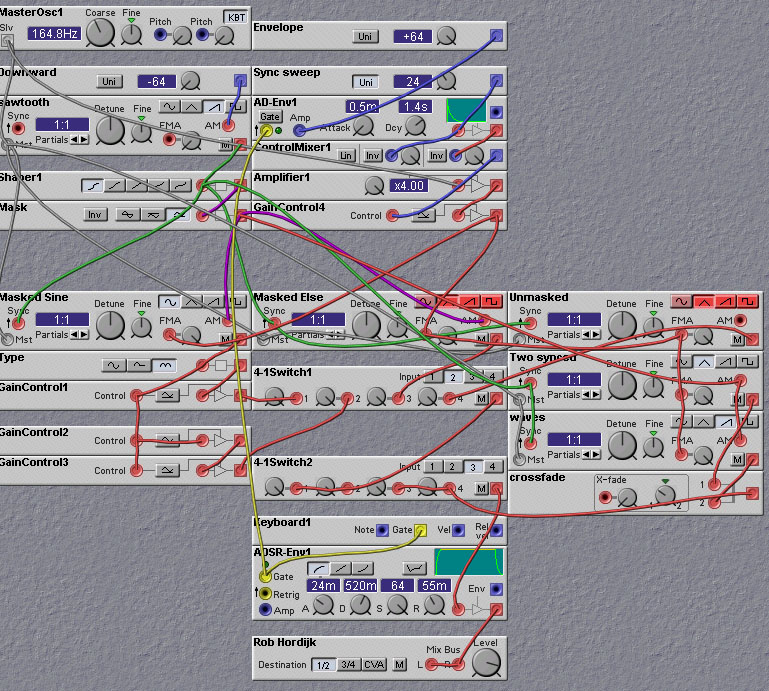Figure 2.2. Patch illustrating a masked hardsync technique (R. Hordijk).

## 2.2 Frequency Modulation

Frequency modulation provides an easy way to alter the harmonic content of a sound. The sounds of many instruments are characterized by the way in which their timbre changes over time. To generate similar sounds using FM is often merely a matter of varying the modulation level appropriately, using envelope generators or LFOs.

The simplest FM techniques feed the output of one sine-wave oscillator, called the modulator, into the frequency modulation input of another, called the carrier. If the two sinewaves have relatively harmonic frequencies, then the resulting waveform out of the carrier will be harmonic as well. The presence and amplitude of the carrier's harmonics is determined by the relative frequency of the carrier and modulator. By adjusting the ratio between these two frequencies we can get quite a range of different harmonic structures.

For example, to emulate Brass instruments using FM, set the frequency of the modulating signal to be the same as that of the carrier. Use an envelope generator to set the modulation level, resulting in the characteristic brightening of the timbre during the attack.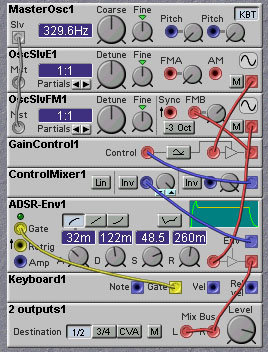Figure 2.3. Patch illustrating a simple FM approach to obtaining a brass-like sound (J. Clark).

Instruments with cylindrical bores, such as the clarinet, have mainly odd harmonics. To obtain this type of spectra we set the frequency of the modulator to be twice that of the carrier. Conversely to the case of brass instruments, the timbre of the clarinet starts out bright then becomes duller during the attack phase. So use an inverted envelope generator to adjust the modulation level.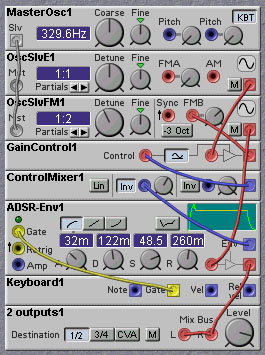Figure 2.4. Patch illustrating a simple FM approach to obtaining a clarinet-like sound (J. Clark).

To model the sound of the Bassoon and similar instruments using FM, set the frequency of the modulator to a sub-multiple of the carrier frequency. This will weaken the strength of the fundamental relative to the higher harmonics. All harmonics (odd and even) are present in these types of instruments, due to the tapered bore.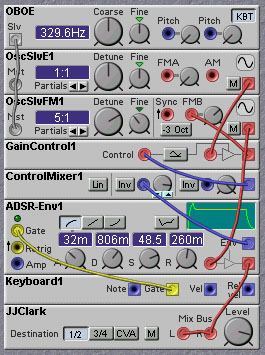Figure 2.5. Patch illustrating a simple FM approach to obtaining an oboe-like sound (J. Clark).

FM synthesis is capable of much more than the simple examples shown here. The output of one carrier oscillator can be used as a modulator waveform as well, and one can create modulation chains, where the spectrum gets more and more complex as one moves down the chain. Modulating the amplitude of the carrier signals in such a chain using envelope generators or LFOs can produce quite expressive and complex time-varying sounds. The following patch shows a three-stage FM chain. The sound is more lively than the simple clarinet patch given above.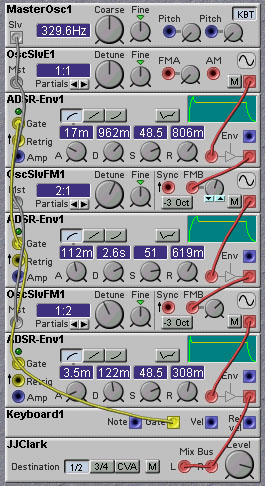Figure 2.6. Patch illustrating the use of multiple modulation stages in an FM patch (J. Clark).

One can use non-sinusoidal waveforms for the carrier and modulators, providing an even greater variety of harmonic spectra. There is no reason one cannot use modulator and carrier frequencies that are non-harmonic. Using these will result in waveforms that have non-harmonic spectra, good for metallic sounds such as bells and gongs. The following two patches illustrate the use of non-sinusoidal modulator and carrier waveforms. The harmonic structure is more complex than in the simple case of sinusoidal waveforms.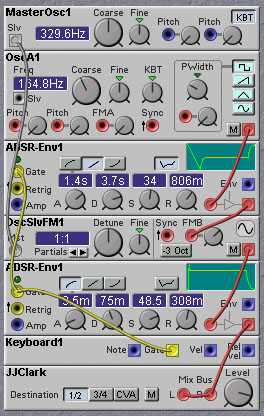Figure 2.7. Patch illustrating non-sinusoidal modulation waveforms in an FM patch (J. Clark).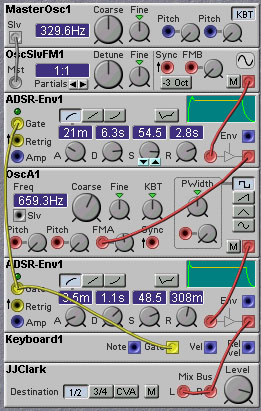Figure 2.8. Patch illustrating non-sinusoidal carrier waveforms in an FM patch (J. Clark).

Feedback is a technique that always produces interesting, if not musical, results, and this is true for FM synthesis as well. One can modulate the first oscillator in a modulation chain with the output of one of the other oscillators (or with its own output, for that matter). The results of applying feedback can be very difficult to analyze mathematically, even in the case where all oscillator frequencies are nominally harmonic. For this reason it is advisable to experiment, and see what comes about. Some general rules can be found, however. First is that a little bit of feedback goes a long way - too much feedback causes instability and even chaotic behaviour. Of course, this might be just what you are looking for! Likewise, feedback from an oscillator output closer to the oscillator being modulated gives a more gentle effect than feedback from an output further down the chain. Inserting time delays into the feedback patch can create very unpredictable shifts in the timbre.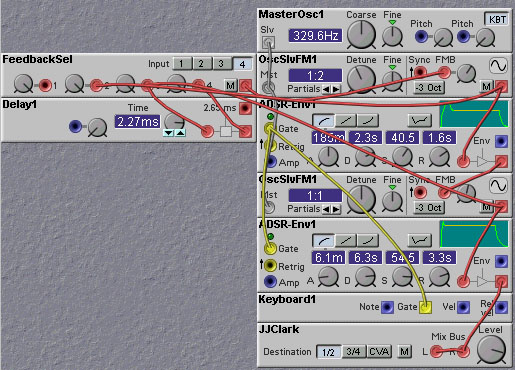Figure 2.9. Patch illustrating the effects of feedback back to the first oscillator in an FM modulation chain (J. Clark).

In the above patch Knob 2 selects the source of the feedback signal, so that you can compare the effects of each. Selecting position 1 gives no feedback, position 2 gives feedback from the first oscillator (i.e. self-modulation of this oscillator), position 3 gives feedback from the second oscillator in the chain, while selecting position 4 gives time delayed feedback from the second oscillator. Play around with the amount of feedback by adjusting the OscSlave1 FM Amount control (Knob 1). If it is set too high a chaotic noisy waveform will be the result.

The oscillator modules on the Nord Modular are specifically designed to permit useful Frequency Modulation synthesis. There are four types of frequency modulation inputs on these modules - "Mst", "Pitch", "A" and "B". The "Mst" and "Pitch" frequency modulation inputs are logarithmic, while the "A" and "B" inputs are linear. The Pitch input is typically used to set the basic pitch of the oscillator, for example, from a keyboard note value. The "Mst" input is usually connected to the "Slv" output of a master oscillator.

To understand the difference between the different input types, consider a typical approach to FM synthesis, where we have two sources determining the frequency of an oscillator. The oscillator adds these two sources together to determine the actual frequency of the oscillator. Typically, one source value is a `stable' one that controls the basic pitch of the waveform (e.g. the "note" being played). The other source is some waveform that varies at an audio rate. The `stable' input should be logarithmic (=non-linear) to accomodate a keyboardvalue on the NM. If the other modulation input (the one connected to the audio rate signal) is also logarithmic the waveform shape, and therefore the timbre, changes if the pitch is increased. A low pitch will be relatively more modulated (have more harmonics) than a high pitch. Sometimes this is desirable, for example when making a fat bass sound, but it makes it not very useful for synthesizing a `physical instrument'-like sound. When modulating with a linear modulating input the harmonic content will remain stable over the complete pitch range.

But what if we selfmodulate a sine-oscillator with the FMA-input? Opening the FMA-knob will not only change the timbre, but also the pitch of the sound, since the average value of the sine output is non-zero. This means that the pitch of the sound will no longer track the note value being fed into the logarithmic pitch input. Not a good thing for emulating standard instruments! Of course, you might be looking for just that off-key type of sound... The FMB input is designed to prevent such a shift in pitch when a waveform with a non-zero average value is used as a frequency modulation source. This input is designed to mimic the form of frequency modulation used on the Yamaha FM-synthesizers. On these synthesizers, such as the DX-7, the pitch doesn't change when selfmodulating. This is because Yamaha used `phase modulation' rather than frequency modulation. In this technique, it is not the frequency of the oscillator that is modulated but it rather the phase of the wave. If the modulating waveform has a non-zero average value, there will only be an (inaudible) shift in phase, and not a shift in pitch. On the Nord Modular we can obtain Yamaha-like FM patches by means of the FMB input. The FMB input does not directly modulate the phase of the oscillator. Instead, the FMB input is passed through a highpass filter (inside the module) and the filtered result is used to frequency modulate the oscillator. The highpass filter removes the DC component of the modulating signal, thereby ensuring that the average value is zero. Hence no pitch shift will occur. Also, consider that a highpass filter acts like a signal differentiator for signals whose frequencies are on the low frequency cutoff slope region of the filter. Frequency modulation with the derivative of a signal is similar to phase modulation with the signal itself (since frequency is the time derivative of phase). Thus, signals applied to the FMB input result in something similar to phase modulation, as in the Yamaha systems. For modulating signals with frequencies above the cutoff frequency of the highpass filter, the modulation will be of frequency and not of phase, so the character of the sound created by the modulation with high frequencies will be different than that for modulation with low frequencies. One might want to lowpass filter the modulating waveform to eliminate the high frequency components that will adversely affect the sound.

Due to the great success and popularity of the Yamaha DX-7 FM synthesizer, there has been much written on the programming of FM synths. There is also a large number of patches available for study. Wout Blommers has created a vast collection of Nord Modular patches based on Yamaha DX-7 patches. These can be found, along with additional information about FM synthesis in the section on Emulating Classic Synths. Rob Hordijk has also written a nice tutorial on FM synthesis which can be accessed at http://www.clavia.se/nordmodular/Modularzone/FMsynthesis.html.

## 2.3 Wave Shaping

The timbre associated with a given waveform is determined primarily by the harmonic content of the waveform. One can create new harmonics by passing the waveform through a nonlinear element, normally referred to as a waveshaper. The principal Nord Modular modules used for waveshaping are the Signal Shaper, Wave-Wrapper, Quantizer, Diode, Clip, and Overdrive modules. Note that the Ring Modulator is not a nonlinear element, but is (bi-)linear. Nonlinearities can be created with combinations of other modules (such as using the gain control and ring modulator modules to multiply a signal by itself) or by (mis-)using the Logic Modules.

Nonlinear elements are defined by the dependance of the element's output on the amplitude of the input. In a linear system, if one increases the input amplitude by a given amount, the output amplitude is also increased by this amount. The shape of the output is not changed due to the increase in input amplitude, just its amplitude. In a nonlinear system this is not the case. It is therefore recommended to apply the nonlinear element after a VCA in a signal chain. As the VCA gain is altered, for example with an envelope or LFO, the amplitude of the waveform is changed. Since the level of the harmonics created by the nonlinear element depend on the amplitude of its input, applying the nonlinearity to the output of the VCA will result in time-varying harmonics. If the nonlinearity was applied before the VCA, the harmonic levels would be static and uninteresting. Likewise you should apply a filter after a nonlinear element rather than before (although interesting effects can be obtained by feeding the output of a resonant filter into a nonlinear element). If you are mixing together different waveforms, it is best to apply the nonlinearity before the mixing. This keeps the harmonics pure. If you are using VCAs you will need one for each waveform. Of course, interesting results can be obtained with applying nonlinearities after mixing, but the result is harder to control and the sound can get muddy if you are not careful.

The following patch illustrates the use of nonlinear elements in waveshaping.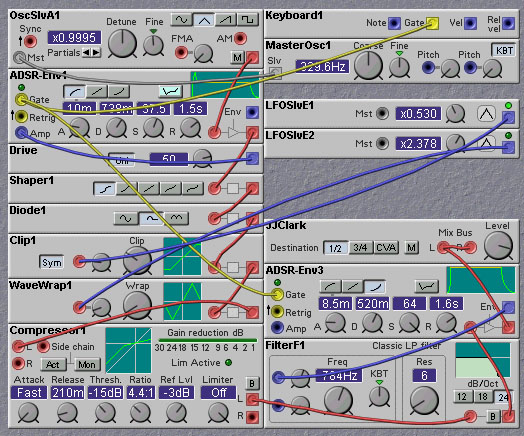Figure 2.10. Patch illustrating waveshaping with nonlinear elements (J. Clark).

Note the use of a compressor after the nonlinear elements. The reason for this is to even out the changes in overall amplitude that can result from the application of the nonlinearities. Remember, the purpose of the nonlinear elements here is to modify the shape of the waveform, but not its amplitude. You want to use a VCA/Envelope Generator after the compressor to provide the dynamics for the patch.

The waveforms that you use as input to the nonlinearities are not too crucial but they do make some difference. In particular, you should avoid using pulse or square waves as input, as their shape is not affected by nonlinear elements, only their amplitude. In this regard, they are much like sine waves with respect to linear filtering operations - the shape of a sine wave is not affected by linear filtering, only its amplitude. In mathematical jargon, sine waves are eigenfunctions of linear time invariant systems (which is a fancy germanic way of saying that the shape of sine waves are not altered by linear filters) and pulse (two-level) waves are eigenfunctions of (memory-less) nonlinear mappings. Typically triangle waves or sawtooth waves are preferable, as their linear slopes makes predicting the effect of nonlinear operations easier than for other waveforms. In some synthesizers, many waveforms are derived from easy to produce triangle waves. For example, one can use a nonlinear element that has a gradual decrease in gain with amplitude to round off the corners of the triangle waves, producing an approximation to a sine wave. This is also an example of a case where application of a nonlinear process results in fewer harmonics than exist in the input!

## 2.4 Vector Synthesis

Vector Synthesis is a waveform modification technique that was developed by Sequential Circuits, and first appeared in their Prophet VS synthesizer. Vector synthesis involves the crossfading of two (or more) waveforms under user or program control. The Prophet VS used four waveforms, visualized as lying on the four corners of a square, and the performer used a 2-D joystick to move within the square. When the joystick moves close to one of the corners, the waveform associated with that corner becomes dominant and the other waveforms are attenuated. When the joystick is in the interior of the square, a linear combination of the waveforms is produced. In the Prophet VS a joystick was used to scan the four waveforms. The following patch illustrates the vector synthesis technique.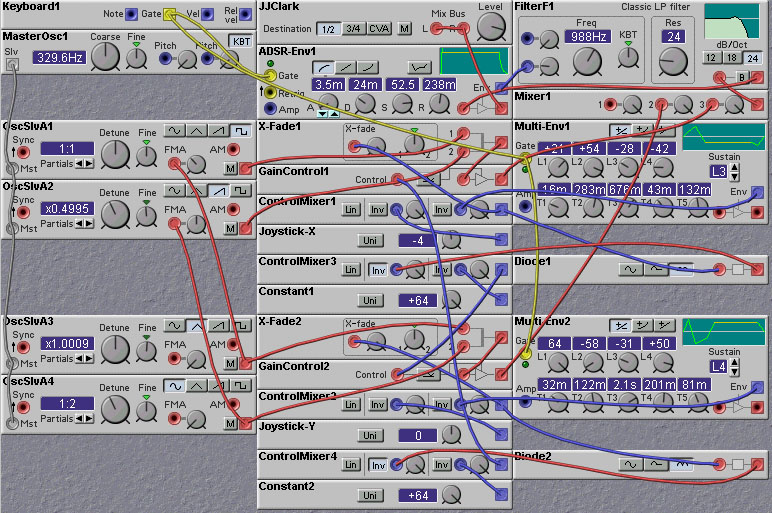Figure 2.11. Vector synthesis patch (J. Clark).

The crossfading between the four oscillators is done via the mathematical relation:

F = ([A*(64-X)+B*(64+X)]*(64-|Y|)+[C*(64+Y)+D*(64-Y)]*(64-|X|))/128/64

where A,B,C,D represent the four waveforms and X and Y represent the two crossfade signals, which range from -64 to 64. The absolute value operation (|X| and |Y|) is implemented with the diode modules. This function has the desired properties that for X=64, Y=0, F=B; for X=-64, Y=0, F=A; for X=0, Y=64, F=C; for X=0, Y=-64, F=D; and for X=Y=0, F=(A+B+C+D)/2. Other crossfade schemes that have these properties are possible, of course.

One can iterate the vector synthesis approach, so that the basic waveforms used in these approaches are themselves vector synthesized waveforms. If you have enough computational resources, you can carry this iteration deeper and deeper, leading to fractal waveforms in the limit. The patch shown in the following figure implements a 2-level vector synthesis algorithm, using 16 oscillators (4 groups of 4 oscillators) with five 2-D waveform crossfade controls (4 to do the second level waveforms, and one to do the top level waveform). LFOs and envelopes are used to generate the crossfade controls, but, of course, any source of control signal could be used.

## 2.5 Wave Sequencing

Wave sequencing is a descendent of vector synthesis. Wave sequencing used up to 255 different waveforms rather than the four of vector synthesis. In wave sequencing various waveforms from the set were sequentially played back and crossfaded, according to some control signal. A precursor to wave sequencing was found in the PPG Wave synthesizer, which used a wavetable of 32 single cycle waveforms which were selected by an index controlled by an envelope or LFO. The waveforms in the set were all related, with little difference between waveforms in adjoining locations in the wavetable. One of the first instruments to employ wave sequencing was the Korg Wavestation. In this machine, the wavetable could have up to 255 different samples. These samples could be wildly different, and were not neccessarily of single cycle waveforms. This flexibility comes at a cost, however, and that is the increased complexity of designing suitable control sequences. One can adjust the rate of crossfading from one wave to the next, as well as the amount of time spent on each waveform. And then there is the agonizing choice of which waveforms to use, and in what order!

In the following patch a wave sequencing algorithm is implemented to generate a single complex waveform. In this patch the order of the waveforms is specified, as well as the amplitude and tuning of each step. The duration of each step is also specified.

One of the useful ways to work with wave sequencing is to arrange the harmonic content (and the crossfade rate) to achieve a given effect. For example, one can place bright sounding waveforms, with fast crossfade rates, early in the sequence, with darker waveforms, with slow crossfades later in the sequence. This will give the typical early-bright-late-dull sound characteristic of many natural instruments. This arrangement is implemented in the patch below. Of course, with the Nord Modular, the range of waveforms that we have to work with is much more limited than on a machine like the Korg WaveStation, so don't expect results to be as spectacular. This patch also lacks some effects that are found on the Wavestation, such as moving backwards and forwards through a set of waveforms, and the specification of a controllable starting point.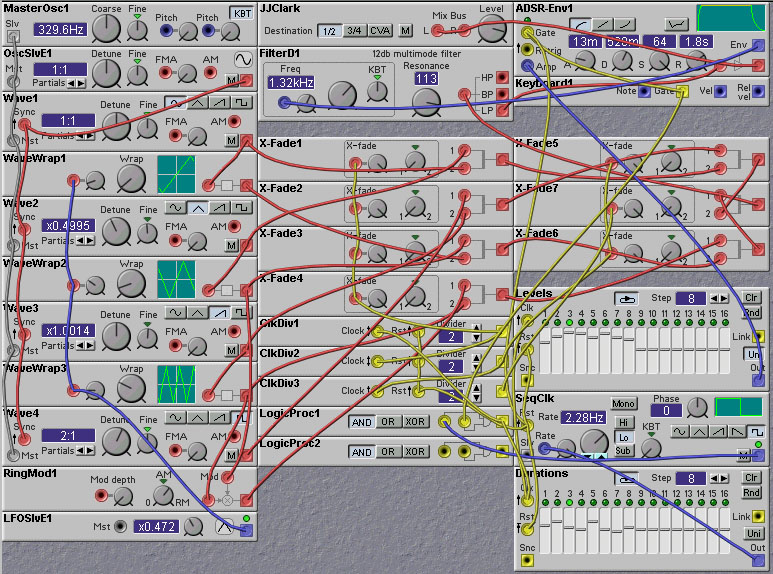Figure 2.12. Wave Sequencing patch (J. Clark).

Note the use of oscillator sync in the above patch. This is to model the fact that in wavetable instruments such as the Prophet VS and Korg Wavestation, the generation of the waveforms is implemented by scanning of waves in the wavetable, and crossfading between waveforms is acomplished by interpolation between different wavetable entries. Thus each waveform is effectively synchronized, and start at the same point.

In the patch above a 3-bit binary counter is constructed from clock divider modules. This counter controls the crossfading of the waveforms in a binary tree. Unfortunately, this approach requires that the crossfades be done quickly, so there is no possibility for smoothing of the crossfades. The next patch, designed by Rob Hordijk, uses a different scheme for crossfading, which permits smoothing of the crossfades. The amount, or smoothness, of the crossfading from one step to the next can be set by the programmer. An abrupt crossfade will give more high frequency components than a smooth crossfade.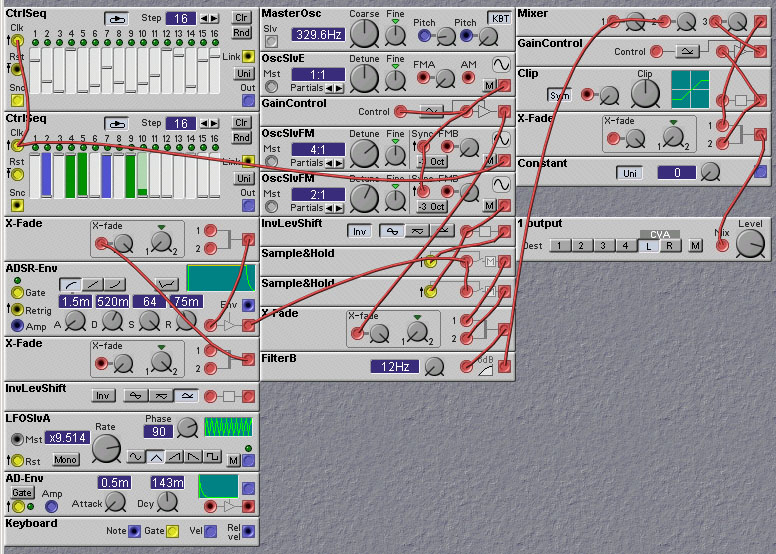Figure 2.13. Wave Sequencing patch that allows smoothing of crossfades from one wave to the next (R. Hordijk).

The crossfader module can be used to interpolate between a current and a previous output of a control sequencer to create a smooth wavetable oscillator. It proved that using the first quadrant of a sine^2 function to interpolate the transitions sounds the most smooth. Nice, as now only three DSP-cheap sinewave slaveoscs, a gain controller, two S&H's, an inverter, the crossfade mixer and a control sequencer to hold the table are needed to do the trick.

Using two (or more) control sequencers allows for fades between waveforms. Its quite a cheesy sound, so to make the sound a bit grittier a fuzz is added.

The control sequencers give up around E5 or so, from there up the patch starts to freak out. Which can be quite nice when e.g. controlled from an external sequencer.

A useful technique for obtaining interesting timbres involves the use of crossfaders being modulated at audio rates. A crossfader combines two input signals, s_1(t) and s_2(t) through a weighted sum controlled by a modulating signal, m(t), as follows:

s_out(t) = K*[(1+m(t))*s_1(t) + (1-m(t))*s_2(t)] = K*[m(t)*s_1(t) - m(t)*s_2(t) + s_1(t) + s_2(t)]

This is equivalent to amplitude- (or ring-) modulation of each input signal by the modulating signal, inverting one, and summing the results with the unmodulated inputs. One could get the same timbre by using two ring-modulators and a mixer module. The advantage of using the crossfader approach is that it is slightly more efficient, and is often easier to understand conceptually the resulting waveform. This is especially true when the two input signals coming from synchronized oscillators and have frequencies that are integer multiples of the modulating signal's frequency, and when the modulating signal is a square wave. In this case alternate cycles of the output waveform will be copies of single cycles of the two input waveforms. Some examples of the resulting waveforms are shown in the figure below, for the case where one input signal has a triangle waveform and the other a sawtooth.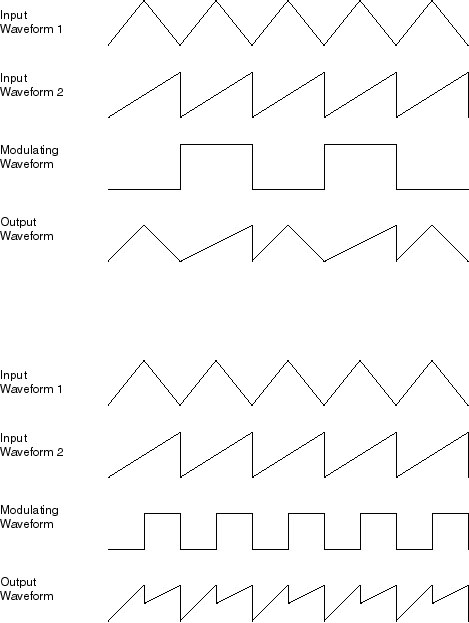Figure 2.14. Two examples of waveform modification using audio-rate crossfading.

A patch that implements this simple audio-rate crossfading idea is given below. The two input oscillators are synced, so you can alter the harmonic structure by adjusting the frequency of the synced oscillator. Try changing the oscillator waveforms to hear the sound produced in the various cases.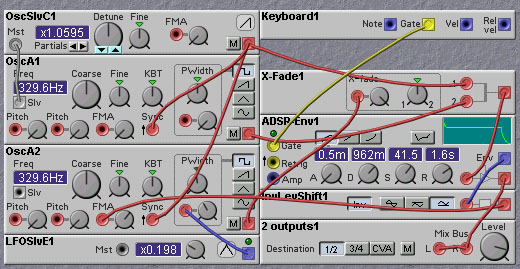Figure 2.15. Audio-rate crossfading patch (J. Clark).

## 2.7 Wave Terrain Synthesis

Wave Terrain synthesis is an extension of wavetable synthesis to the use of 2-dimensional wavetables. Now a true 2-D wavetable would require a very large amount of memory, so wave terrain synthesis seeks to save on memory by defining the 2-D wavetable with a surface, called the terrain, defined by a mathematical operation called the terrain function. Two 1-D wavetables are then used to define the coordinates on this 2-D surface. If we refer to the two 1-D waves as x(t) and y(t), where t is the wavetable index, then the output of the terrain synthesis is obtained by acting on x(t) and y(t) by the terrain function, z(x,y). The signals x(t) and y(t) define an Orbit in the terrain, which selects which values to be output.

Wave Terrain synthesis is described in the book Computer Music Tutorial, by Curtis Roads, pp.163-167. In an example of its usage, he implements the following terrain function, and scans it with two signals, x and y:

z = (x - y) * (x - 1) * (x + 1) * (y - 1) * (y + 1)

A Nord Modular patch based on this example has been created by Chet Singer, and is shown below.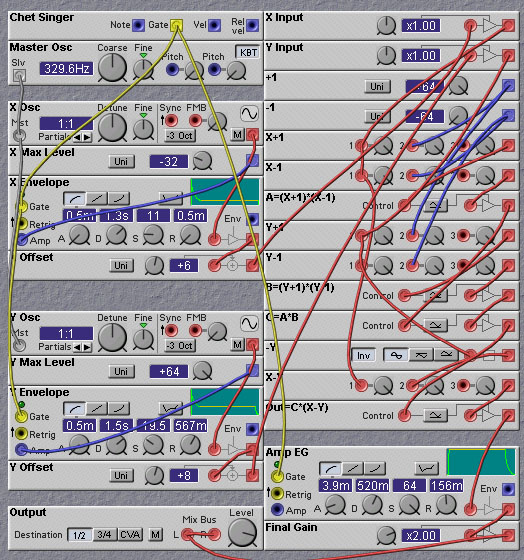Figure 2.16. A simple Wave Terrain patch (C. Singer).

In this patch the two 1-D waves which define the orbit on the terrain function are enveloped sine waves. The resulting orbit traces out two circular paths connected by a spiral on the terrain surface. At the beginning of the envelope, the path follows the outer circle, then quickly moves to the inner circle during the sustain phase. Upon the release of envelope the orbit moves to the center of the circular paths and stays there. The circles are offset somewhat from the center of the terrain (see the "Offset" control modules in the patch). This adds some assymmetry to the sound and makes it more interesting.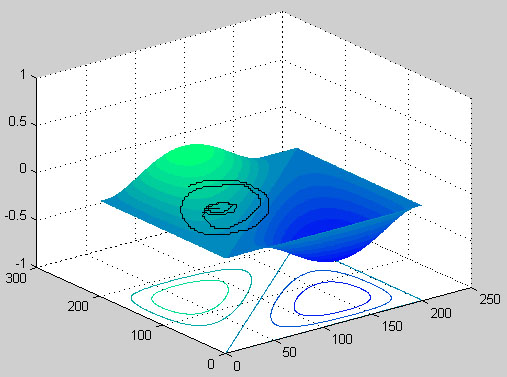Figure 2.17. The Terrain function used in the above patch, with a typical circular orbit.

## 2.8 VOSIM

For a description of the VOSIM technique, with Nord Modular patch examples, read Rob Hordijk's tutorial on the subject, http://www.clavia.se/nordmodular/Modularzone/VOSIM.html.

## 2.9 FOF (Fonction d'Onde Formantique) Synthesis

FOF synthesis is a technique for producing waveforms with a specific spectral shape. It was developed by Xavier Rodet at IRCAM. Similar to the VOSIM technique, sounds are produced from a sequence of excitation pulses in the form of enveloped sine waves. The rate of generation of the excitations specifies the fundamental frequency of a formant region. Harmonics of this fundamental are also present, and their amplitude is determined by the shape of the envelope that is applied to each sinusoid in formaing the excitation pulses. The shorter this envelope, the more gradual the falloff in harmonic amplitude in the frequency domain. Lengthening the envelope, on the other hand, reduces the harmonic amplitudes quickly in the the frequency domain, narrowing the width of the formant region. Other details of the local envelope shape, such as the rise and fall rates, affect the shape of the harmonic amplitude curves in subtle ways, allowing for precise control of the harmonic structure. Of course, the shape of the local envelope can be changed over time, permitting dynamic timbres to be produced. Typically, many such excitation waveforms are added together, each providing a different formant region. In this way vowel-like vocal sounds can easily be created, although non-vocal sounds are also possible.

The following patch, designed by Pere Villez, implements a basic FOF synthesis system. A slightly more complex patch, FoFGen01Cello02.pch by the same designer, shows the ease with which FOF synthesis can model string instruments.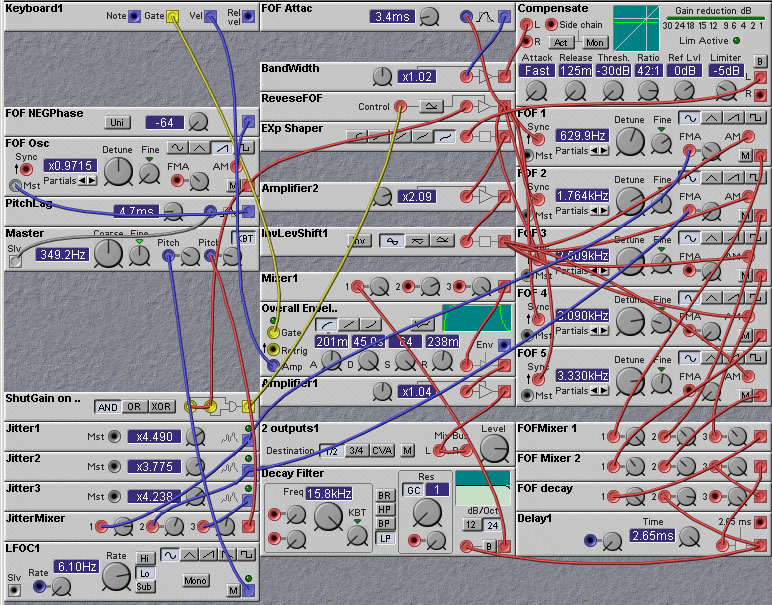Figure 2.18. A FOF patch (P. Villez).

## 2.10 Granular Synthesis

Granular synthesis is similar in many ways to the VOSIM and FOF synthesis techniques described above. A grain is a short piece of a waveform (which can come from any source, but is typically a sample stored in a wavetable) which has a short-time envelope applied to it. Each grain is generally 10-100 milliseconds in length. Many individual grains are combined with various relative timings and overlaps to create a composite "cloud" of sound. The individual grains can be modified by altering their envelope (lengthening or shortening, changing the rise and fall time, etc) and changing the frequency of the grain waveform (by changing the scan rate of the wavetable, for example). The relative positions of the grains in the cloud can be altered as well. All of these changes can either be deterministic or random.

The total number of grains, the grain pitch, the inter-grain interval (or overlap if interval is negative), grain envelope shape (attack and decay), and grain length are commonly altered in a granular synthesis algorithm.

The following patch, by Pelle Dahlstedt, illustrates how Granular synthesis can be implemented on the Nord Modular.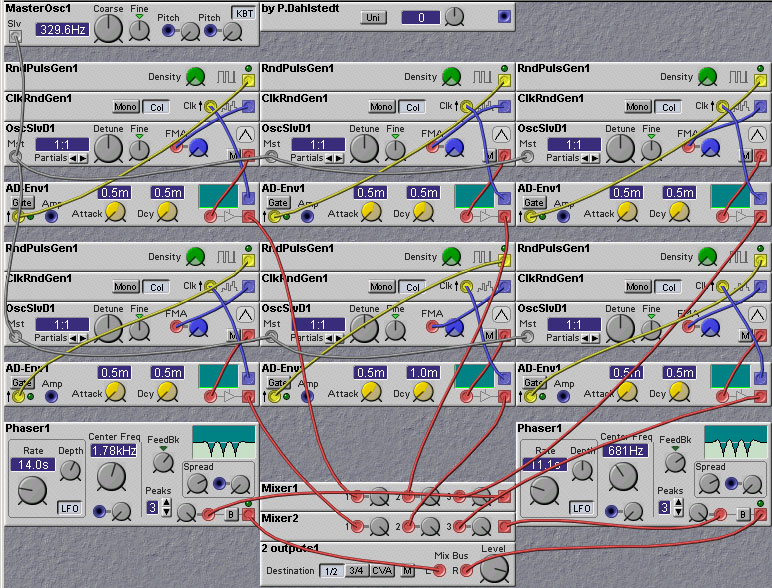Figure 2.19. A Granular synthesis patch (P. Dahlstedt).

[Prev (Introduction)][Next (Filter Techniques)][Table of Contents](c) James Clark 2003